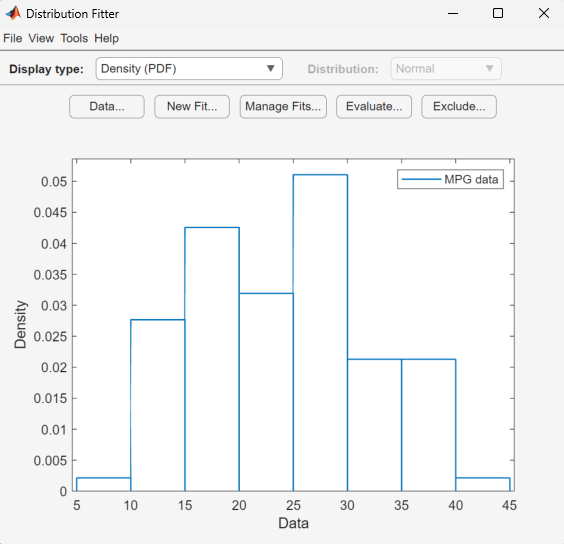Documentation

# dfittool

Open Distribution Fitter app

The `distributionFitter` function was introduced in R2017a as a replacement for the `dfittool` function. Both functions continue to work to start the Distribution Fitter app.

This page contains programmatic syntax information for the Distribution Fitter app. For general usage information, see Distribution Fitter.

## Syntax

``dfittool``
``dfittool(y)``
``dfittool(y,cens)``
``dfittool(y,cens,freq)``
``dfittool(y,cens,freq,dsname)``

## Description

````dfittool` opens the Distribution Fitter app, or brings focus to the app if it is already open. ```

example

````dfittool(y)` opens the Distribution Fitter app populated with the data specified by the vector `y`.```

example

````dfittool(y,cens)` uses the vector `cens` to specify whether each observation in `y` is censored.```
````dfittool(y,cens,freq)` uses the vector `freq` to specify the frequency of each element of `y`.```
````dfittool(y,cens,freq,dsname)` creates a data set with the name `dsname`, using the data vector, `y`, censoring indicator, `cens`, and frequency vector, `freq`. ```

## Examples

collapse all

Load the `carsmall` sample data.

`load carsmall`

Open the Distribution Fitter app using the `MPG` miles per gallon data.

`dfittool(MPG)`The Distribution Fitter app opens, populated with the `MPG` data, and displays the density (PDF) plot. You can use the app to display different plots and fit distributions to this data.

Load the sample data.

`load(fullfile(matlabroot,'examples','stats','lightbulb.mat'))`

The first column of the data contains the lifetime (in hours) of two types of light bulbs. The second column contains information about the type of light bulb. 1 indicates fluorescent bulbs, and 0 indicates the incandescent bulb. The third column contains censoring information. 1 indicates censored data, and 0 indicates the exact failure time. This is simulated data.

Open the Distribution Fitter app using the first column of `lightbulb` as the input data, and the third column as the censoring data. Name the data `lifetime`.

`dfittool(lightbulb(:,1),lightbulb(:,3),[],'lifetime')`

To open the Data dialog box, click . In the Manage data sets pane, click to highlight the `lifetime` data set row. Finally, to open the View Data Set dialog, click . The lifetime data appears in the second column and the corresponding censoring indicator appears in the third column.## Input Arguments

collapse all

Input data, specified as an array of scalar values or a variable representing an array of such values.

Data Types: `single` | `double`

Censoring indicator, specified as a vector of 0 and 1 values. The length of `cens` must be equal to the length of `y`. If `y(j)` is censored, then `(cens(j)==1)`. If `y(j)` is not censored, then `(cens(j)==0)`. If `cens` is omitted or empty, then no `y` values are censored.

If you have frequency data (`freq`) but not censoring data (`cens`), then you must specify empty brackets (`[]`) for `cens`.

Data Types: `single` | `double`

Frequency data, specified as a vector of scalar values. The length of `freq` must be equal to the length of `y`. If `freq` is omitted or empty, then all `y` values have a frequency of 1.

If you have frequency data (`freq`) but not censoring data (`cens`), then you must specify empty brackets (`[]`) for `cens`.

Data Types: `single` | `double`

Data set name, specified as a character vector enclosed in single quotes or a string scalar enclosed in double quotes.

If you want to specify a data set name, but do not have censoring data (`cens`) or frequency data (`freq`), then you must specify empty brackets (`[]`) for both `freq` and `cens`.

Example: `'MyData'`

Data Types: `char` | `string`

Download ebook